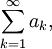# The Ratio Test

Previous: Introduction to the Ratio Test

Next: The Ratio Test Flowchart

The Ratio Test
To apply the ratio test to a given infinite serieswe evaluate the limitThere are three possibilities:

• if L < 1, then the series converges
• if L > 1, then the series diverges
• if L = 1, then the test is inconclusive

The proof of this test is relatively long, and as such is provided in an appendix on the Proof of the Ratio Test.

### Observe Carefully: The Test Can Yield No Information

Before moving on, note that in the case that L = 1, the test yields no information. Applying the ratio test to the harmonic seriesyieldsBecause the limit equals 1, the ratio test fails to give us any information.

But the harmonic series is not a convergent series, so in the case where L = 1, other convergence tests can be used to try to determine whether or not the series converges.

Previous: Introduction to the Ratio Test

Next: The Ratio Test Flowchart